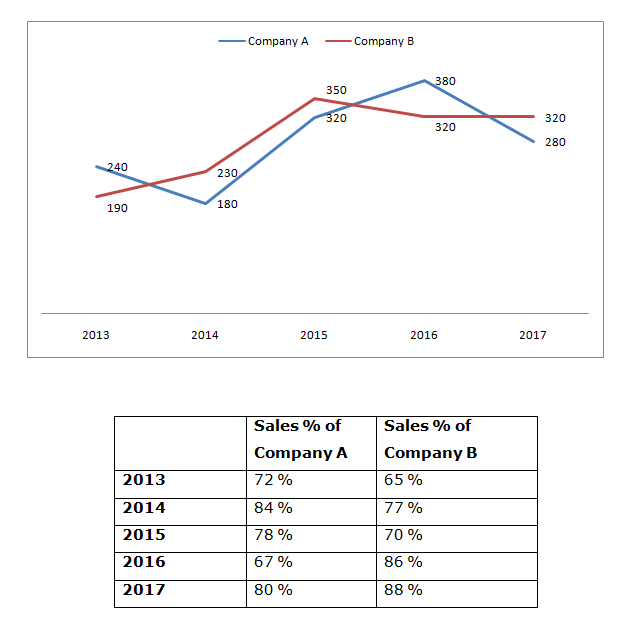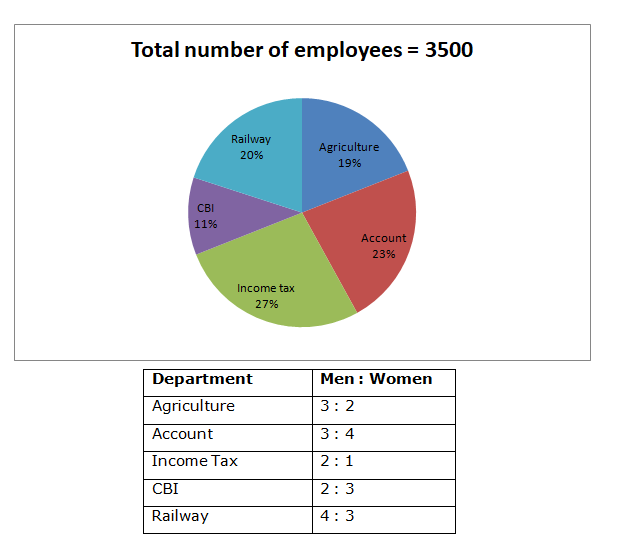# Quantitative Aptitude Questions (Data Interpretation) for SBI Clerk/PO Prelims 2018 Day-146

Dear Readers, SBI is conducting Online Examination for the recruitment of Clerical Cadre and probationary officer. To enrich your preparation here we have providing new series of Data Interpretation – Quantitative Aptitude Questions. Candidates those who are appearing in SBI Clerk/PO Prelims Exams can practice these Quantitative Aptitude average questions daily and make your preparation effective.

[WpProQuiz 2603]

Click “Start Quiz” to attend these Questions and view Solutions

Directions (Q. 1 – 5) study the following information carefully and answer the given questions:

Following line graph shows the total production (In tonnes) of company A and B in different years and the table shows the percentage of sales of company A and B.1. Total production of company A in the year 2013 and 2015 together is approximately what percentage of total production of company B in the year 2014 and 2016 together?
1. 85 %
2. 110 %
3. 102 %
4. 125 %
5. 75 %
1. Find the difference between the total sales of company A in the year 2014 and 2017 together to that of total sales of company B in the year 2015 and 2016 together?
1. 124 tonnes
2. 137 tonnes
3. 156 tonnes
4. 145 tonnes
5. None of these
1. Find the ratio between the total productions of Company A to that of Company B in all the given years together?
1. 127 : 133
2. 140 : 141
3. 132 : 145
4. 56 : 65
5. None of these
1. Find the average sales value of company B in all the given years together?
1. 56 tonnes
2. 72 tonnes
3. 48 tonnes
4. 34 tonnes
5. None of these
1. In which of the following year, the sales of company A is second highest?
1. 2015
2. 2013
3. 2017
4. 2014
5. 2016

Directions (Q. 6 – 10) study the following information carefully and answer the given questions:

Following pie chart shows the total number of employees working in different government department in a certain state and the table shows the ratio of male and female among them.1. Total number of men employees in Agriculture and Railway department is approximately what percentage of total number of women employees in Account and CBI department?
1. 116 %
2. 92 %
3. 84 %
4. 132 %
5. 145 %
1. Find the total number of women employees in all the given department together?
1. 1784
2. 2126
3. 2858
4. 1572
5. None of these
1. Find the ratio between the total number of men employees in Account and CBI department to that of total number of women employees in Agriculture and Income tax department?
1. 256 : 317
2. 144 : 179
3. 499 : 581
4. 342 : 475
5. None of these
1. Find the difference between the total number of men employees to that of total number of women employees in all the given department together?
1. 356
2. 478
3. 524
4. 642
5. None of these
1. Total number of employees in Income tax and railway department is approximately what percentage more than the agriculture and CBI department?
1. 69 %
2. 57 %
3. 75 %
4. 43 %
5. 81 %

Total production of company A in the year 2013, 2015 together

= > 240 + 320 = 560 tonnes

Total production of company B in the year 2014 and 2016 together

= > 230 + 320 = 550 tonnes

Required % = (560/550)*100 = 102 %

The total sales of company A in the year 2014 and 2017 together

= > 180*(84/100) + 280*(80/100)

= > 151.2 + 224 = 375.2 tonnes

The total sales of company B in the year 2015 and 2016 together

= > 350*(70/100) + 320*(86/100)

= > 245 + 275.2 = 520.2 tonnes

Required difference = 520.2 – 375.2 = 145 tonnes

The total production of Company A in all the given years together

= > 240 + 180 + 320 + 380 + 280 = 1400 tonnes

The total production of Company B in all the given years together

= > 190 + 230 + 350 + 320 + 320 = 1410 tonnes

Required ratio = 1400: 1410 = 140: 141

The total sales value of company B in all the given years together

= > 190*(65/100) + 230*(77/100) + 350*(70/100) + 320*(86/100) + 320*(88/100)

= > 123.5 + 177.1 + 245 + 275.2 + 281.6 = 1102.4 tonnes

Required average = 1102.4/5 = 220.48 tonnes

In 2013 = > 240*(72/100) = 172.8 tonnes

In 2014 = > 180*(84/100) = 151.2 tonnes

In 2015 = > 320*(78/100) = 249.6 tonnes

In 2016 = > 380*(67/100) = 254.6 tonnes

In 2017 = > 280*(80/100) = 224 tonnes

Directions (Q. 6 – 10)

Total number of men employees in Agriculture and Railway department

= > 3500*(19/100)*(3/5) + 3500*(20/100)*(4/7)

= > 399 + 400 = 799

Total number of women employees in Account and CBI department

= > 3500*(23/100)*(4/7) + 3500*(11/100)*(3/5)

= > 460 + 231= 691

Required % = (799/691)*100 = 116 %

The total number of women employees in all the given department together

= > 3500*(19/100)*(2/5) + 3500*(23/100)*(4/7) + 3500*(27/100)*(1/3) + 3500*(11/100)*(3/5) + 3500*(20/100)*(3/7)

= > 266 + 460 + 315 + 231 + 300 = 1572

The total number of men employees in Account and CBI department

= > 3500*(23/100)*(3/7) + 3500*(11/100)*(2/5)

= > 345 + 154 = 499

The total number of women employees in Agriculture and Income tax department

= > 3500*(19/100)*(2/5) + 3500*(27/100)*(1/3)

= > 266 + 315 = 581

Required ratio = 499: 581

The total number of men employees in all the given department together

= > 3500*(19/100)*(3/5) + 3500*(23/100)*(3/7) + 3500*(27/100)*(2/3) + 3500*(11/100)*(2/5) + 3500*(20/100)*(4/7)

= > 399 + 345 + 630 + 154 + 400 = 1928

The total number of women employees in all the given department together

= > 3500*(19/100)*(2/5) + 3500*(23/100)*(4/7) + 3500*(27/100)*(1/3) + 3500*(11/100)*(3/5) + 3500*(20/100)*(3/7)

= > 266 + 460 + 315 + 231 + 300 = 1572

Required difference = 1928 – 1572 = 356

Required % = [(47 – 30)/30]*100 = 57 %

Daily Practice Test Schedule | Good Luck

 Topic Daily Publishing Time Daily News Papers & Editorials 8.00 AM Current Affairs Quiz 9.00 AM Quantitative Aptitude “20-20” 11.00 AM Vocabulary (Based on The Hindu) 12.00 PM General Awareness “20-20” 1.00 PM English Language “20-20” 2.00 PM Reasoning Puzzles & Seating 4.00 PM Daily Current Affairs Updates 5.00 PM Data Interpretation / Application Sums (Topic Wise) 6.00 PM Reasoning Ability “20-20” 7.00 PM English Language (New Pattern Questions) 8.00 PM